Quantity: 0

Total: 0,00

0

Perimeter, area, surface area and volume

Perimeter, area, surface area and volume

This animation presents the formulas to calculate the perimeter and area of shapes as well as the surface area and volume of solids.

Mathematics

Keywords

volume, surface, circumference, area, sphere, pyramid, cylinder, circle sector, circle, triangle, rectangle, square, cone, cuboid, base area, lateral surface, parallelogram, formula, geometry, solid geometry, mathematics

Related items

Scenes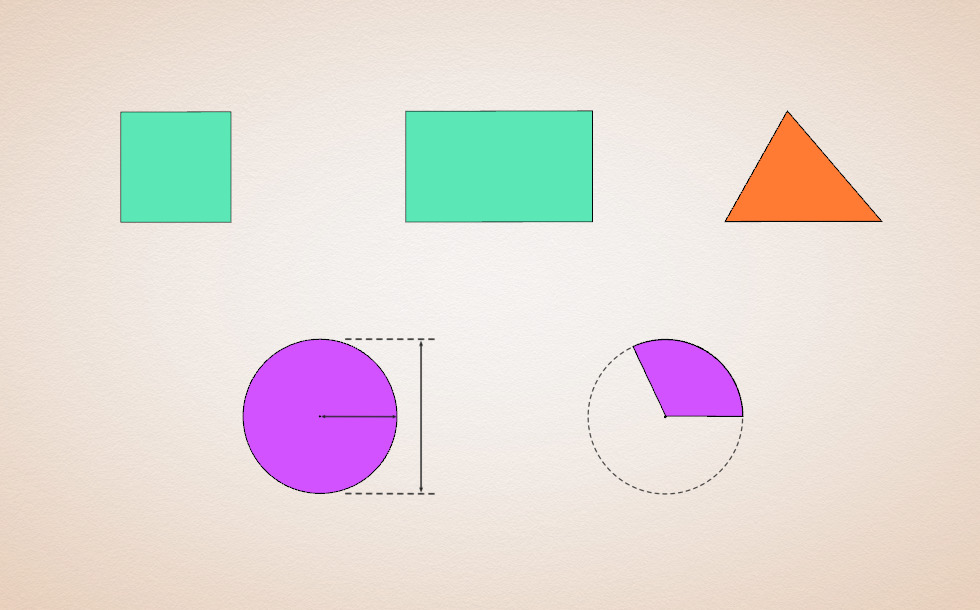Perimeter of shapes

• square
• rectangle
• triangle
• circle
• circular sector
• a
• w
• h
• b
• a
• c
• d
• r
• r
• θ°

A two-dimensional geometric shape is a part of a plane that is enclosed by straight or curved lines, does not contain any holes and remains intact even if one of its points is removed.

The perimeter of a two-dimensional shape is the length of the path that surrounds the shape. It can be calculated by summing the lengths of the lines or curves that enclose the shape.

Since all four sides of a square have equal length, its perimeter is four times the side length.

The opposite sides of a rectangle are of equal lengths, therefore its perimeter is twice the sum of its width and its height.

The perimeter of a triangle is the sum of the three side lengths. In the case of isosceles and equilateral triangles the rule is the same but the calculation is simpler.

The circumference (a special case of perimeter) of a circle is the length of its diameter multiplied by π (pi). (The ratio of the circumference of any circle to its diameter is constant. This mathematical constant is called π.)

The perimeter (or circumference) of a circular sector is the sum of the arc length and the length of the radius multiplied by two (the length of the two radii). The arc length can be calculated from the circumference of the circle, using the ratio of the central angle to the full angle (360°).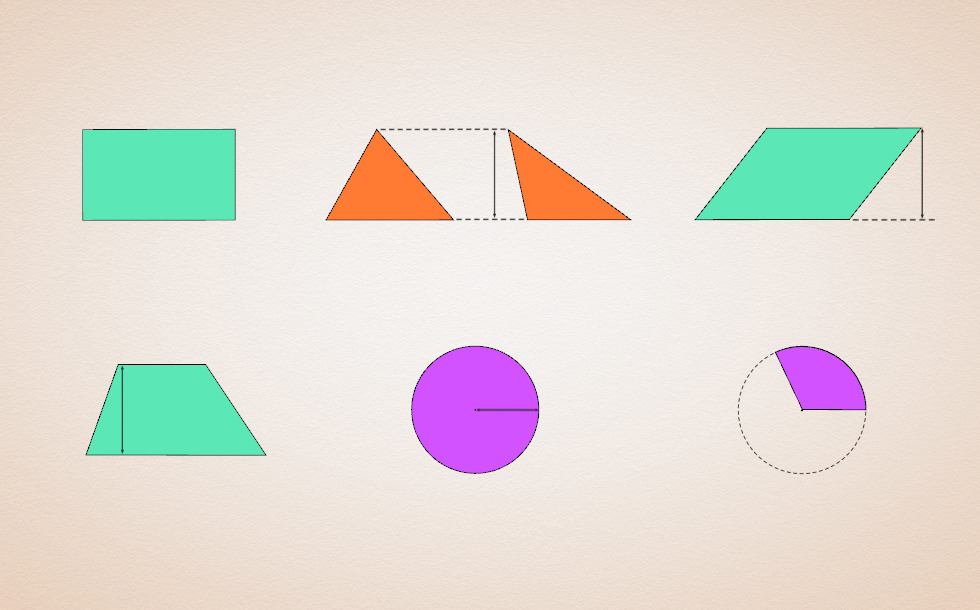Area of shapes

• rectangle
• triangle
• parallelogram
• trapezium
• circular
• circular sector
• w
• h
• b
• b
• h
• b
• h
• b₁
• b₂
• h
• r
• r
• θ°

A two-dimensional geometric shape is a part of a plane that is enclosed by straight or curved lines, does not contain any holes and remains intact even if one of its points is removed.

The area is a function that assigns a positive number to all two-dimensional geometric shapes with the following conditions:

1. The area of the unit square is 1.
2. The area of congruent geometric shapes is equal.
3. If we divide a geometric shape into several parts, the sum of the areas of the parts is equal to the area of the original geometric shape.

The area of a rectangle is the product of its width and height.

The area of a triangle is half the product of its base length and its height. (This formula derives from the formula of the area of the parallelogram.)

The area of a parallelogram is the product of its base length and height.

The area of a trapezium is the product of half the sum of the parallel sides and its height.

The area of a circle can be calculated by multiplying the square of its radius with π (pi).

The area of a circular sector can be calculated from the area of the full circle, using the ratio of the central angle to the full angle (360°).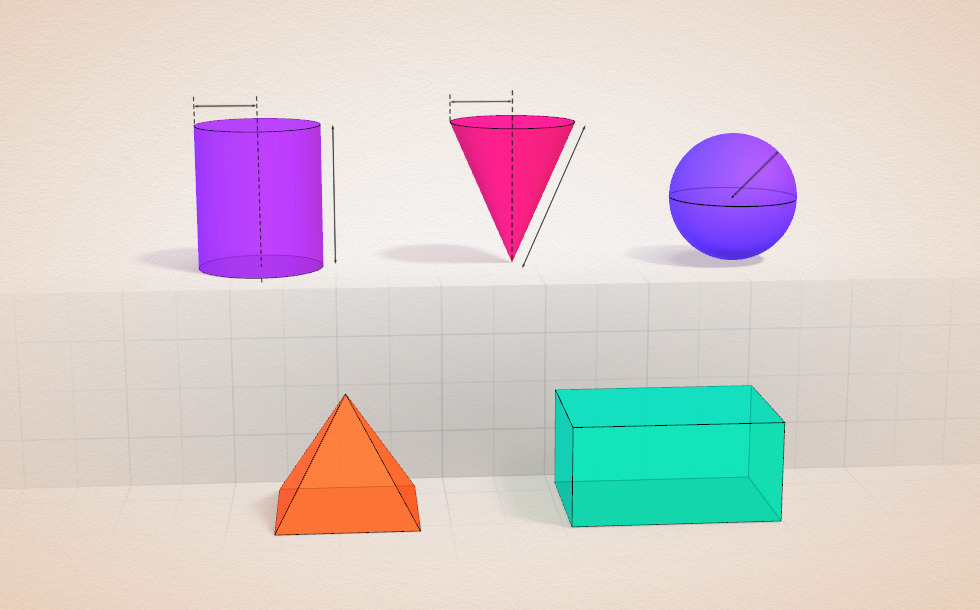Surface area of solids

• cylinder
• cone
• sphere
• pyramid
• cuboid
• r
• h
• r
• g
• r
• l
• h
• w
• r
• h
• r
• h
• r
• h
• l
• h
• w

A geometric solid is a three-dimensional object; an enclosed part of space bounded by polygons or curved surfaces.

The surface area of a cylinder can be calculated by adding the area of its lateral surface to twice the base area. The base of a right cylinder is a circle (or, more precisely, a disk), while its lateral surface is a rectangle, the two side lengths, which correspond to the height of the cylinder and the circumference of the base, respectively.

The surface area of a cone is the sum of the areas of its base and its lateral surface. The base of a right circular cone is a circle (or, more precisely, a disk), while its lateral surface is a circular segment with a radius that corresponds to the generatrix of the cone and the length of the arc corresponds to the circumference of the base of the cone.

The surface area of a sphere can be calculated by multiplying the area of its main circle by four (the radius of the main circle is equal to the radius of the sphere itself.)

The surface area of a pyramid is the sum of the areas of its base and its lateral surface (that is, the sum of the areas of the faces).

The faces of a cuboid are rectangles and the opposite faces are congruent. The surface area of a cuboid is the combined area of the six faces. It can be calculated by multiplying the side lengths of three faces of different dimensions, then adding up the three products and multiplying the sum by two.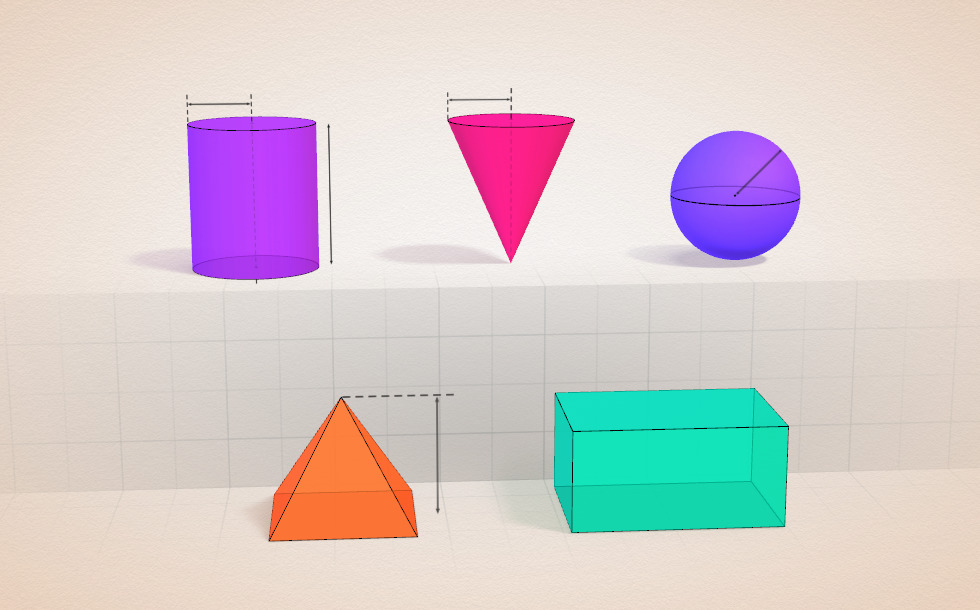Volume of solids

A geometric solid is a three-dimensional object; an enclosed part of space bounded by polygons or curved surfaces.

Volume is a function that assigns a positive number to all geometric solids with the following conditions:

1. The volume of a unit cube is 1.
2. The volumes of congruent solids are equal.
3. If we divide a geometric solid into several parts, the sum of the volumes of these is equal to the volume of the original solid.

The volume of a cylinder is the product of the area of its base and the height. In the case of a right circular cylinder, the base is a circle.

The volume of a cone is the product of the area of the base and the height divided by three. In the case of a right circular cone, the base is a circle.

The volume of a sphere is two thirds the volume of its circumscribed cylinder. The area of the base of the circumscribing cylinder is equal to the area of the great circle of the sphere and the height of the cylinder is equal to the diameter of the sphere.

The volume of a pyramid is one third the volume of a prism that has a congruent shape as its base, and the same height. It can be calculated by dividing the product of the base and the height by three.

The volume of a cuboid is the product of the length, width and height.

Related itemsCuboid

A cuboid is a polyhedron with six rectangular faces.Conic solids

This animation demonstrates various types of cones and pyramids.Cube

This animation demonstrates the components (vertices, edges, diagonals and faces) of the cube, one of the Platonic solids.Sphere

A sphere is the set of points which are all within the same distance from a given point in space.Conic sections

The conic section is a plane curve that is created when a right circular cone is intersected by a plane.Grouping of cuboids

This animation demonstrates various types of cuboids through everyday objects.Platonic solids

This animation demonstrates the five regular three-dimensional (or Platonic) solids, the best known of which is the cube.Ratio of volumes of similar solids

This 3D scene explains the correlation between the ratio of similarity and the ratio of volume of geometric solids.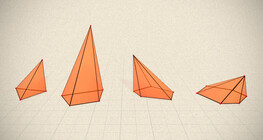Regular square pyramid

A regular square pyramid is a right pyramid with a square base and four triangular faces.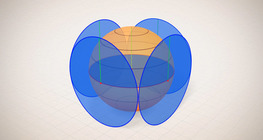Surface area of spheres (demonstration)

The surface of a sphere consists of the set of points which are all at the same distance from a given point in space.Volume and surface area (exercise)

An exercise about the volume and surface area of solids generated from a ´base cube´.Volume of spheres (Cavalieri´s principle)

Calculating the volume of a sphere is possible using an appropriate cylinder and cone.Volume of spheres (demonstration)

The sum of the volume of the ´tetrahedrons´ gives an approximation of the volume of the sphere.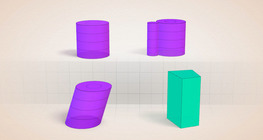Cylindrical solids

This animation demonstrates various types of cylindrical solids as well as their lateral surfaces.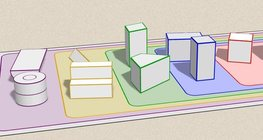Grouping of solids

This animation demonstrates various groups of solids through examples.Net of a cube (exercises)

Not all nets consisting of 6 congruent squares are foldable into cubes.Solids of revolution

Rotating a geometric shape around a line within its geometric plane as an axis results in a solid of revolution.